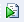Scripting Guide > Scripting Platforms > Example of Scripting a Platform
Publication date: 11/10/2021

# Example of Scripting a Platform

If you are not familiar with JSL, it can be helpful to start by interactively performing your analysis in JMP, and then saving the analysis to a script. JMP creates the script for you, and you can then add to it or modify it as desired, by creating a platform object and sending it messages.

#### Overview

This example takes you through these steps:

#### Launch the Analysis and Save the Script to a Window

1. Select Help > Sample Data Library and open Big Class.jmp.

This fictional data set contains the names, ages, sex, heights, and weights for 40 students.

2. Select Analyze > Fit Y by X.

3. Select height and click Y, Response.

4. Select age and click X, Factor.

5. Click OK.

A Oneway plot of students’ height by age appears.

6. Click the Oneway Analysis red triangle and select the following options:

Means/Anova

Compare Means > Each Pair, Student’s t

7. Click the Oneway Analysis red triangle and select Save Script > To Script Window.

Here is the resulting script:

`Oneway(`
`	Y( :height ), X( :age ),`
`	Each Pair( 1 ),`
`	Means( 1 ),`
`	Mean Diamonds( 1 )`
`);`

Notice the following:

The script begins with a call to the Oneway() function, which returns a Oneway object.

The parentheses after the Oneway() function contain arguments that tell the Oneway function how to make the object.

The first two arguments, Y and X, are required at launch.

The next three arguments are optional: Each Pair, Means, and Mean Diamonds.

When you selected the Each Pair, Student’s t option, the Each Pair feature was turned on.

When you selected the Means/Anova option, the Means and Mean Diamonds features were turned on.

The scripting equivalent of turning an option on or off is the Boolean argument 0 (off) or 1 (on).

#### Add a Platform Reference Object and Send Messages

1. (Optional) Right-click in the script and select Show Embedded Log.

Output that appears in the log is now easily visible below the script.

2. Choose a JSL variable to remember the Oneway platform object.

In this example, use the name oneObj:

`oneObj = Oneway(`
`	Y( :height ),`
`	X( :age ),`
`	Each Pair( 1 ),`
`	Means( 1 ),`
`	Mean Diamonds( 1 )`
`);`

This gives you a way to address the platform object and send messages to it.

3. Click Run Script.

This creates the Oneway object and sets the variable oneObj. Now, send a message to tell the platform to turn on the Unequal Variances report.

4. At the end of the script, add the following line: oneObj << Unequal Variances(1), as shown here:

`oneObj = Oneway(`
`	Y( :height ),`
`	X( :age ),`
`	Each Pair( 1 ),`
`	Means( 1 ),`
`	Mean Diamonds( 1 )`
`);`
`oneObj << Unequal Variances( 1 );`

5. Highlight the Unequal Variances line of JSL and click Run Script.

Tip: You can also press Ctrl+R to run a highlighted line of JSL.

At the bottom of the report window, the Tests That the Variances Are Equal report now appears. Now, suppose that you want to see only that report (and the graph), and you want to close the other reports.

6. Add the following lines to the script:

`rep = Report( oneObj );`
`rep["Oneway Anova"] << Close( 1 );`
`rep["Means Comparisons"] << Close( 1 );`

The Report() function returns the report object for the Oneway platform, and stores a reference to the report in the JSL variable called rep. You can send messages or perform actions on the report object, such as closing specific report outlines.

7. Highlight these last 3 lines of the script and click Run Script.

In the report window, the Oneway Anova and the Means Comparisons reports are now closed. Only the initial graph and the Tests that the Variances are Equal report (which includes the Welch’s Test report) appear.

If you close the Big Class.jmp sample data table at this point and then try to run the script, you are prompted to open a data table. A best practice is to precede the call to the Oneway platform with an Open() function to open the associated data table each time the script is run.

#### Add a Data Table Reference and Save the Script

1. Add the following line as the first line in the script:

`dt = Open( "\$SAMPLE_DATA/Big Class.jmp" );`

If the data table is not open when the script is run, this line opens the associated data table.

Your finished script should look like this:

`dt = Open( "\$SAMPLE_DATA/Big Class.jmp" );`
`oneObj = Oneway(`
`	Y( :height ),`
`	X( :age ),`
`	Each Pair( 1 ),`
`	Means( 1 ),`
`	Mean Diamonds( 1 )`
`);`
`oneObj << Unequal Variances( 1 );`
`rep = Report( oneObj );`
`rep["Oneway Anova"] << Close( 1 );`
`rep["Means Comparisons"] << Close( 1 );`

Once you complete your script, save it for future access.

2. From the script window, select File > Save As. Enter a name for your script and save it to any directory.